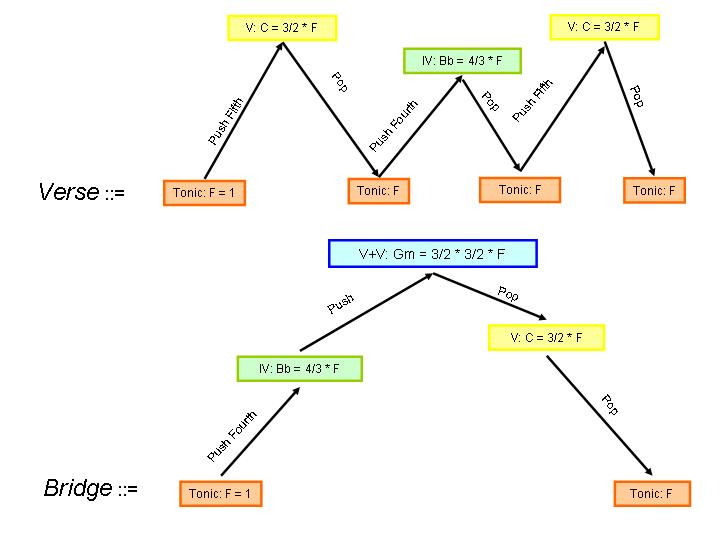[an error occurred while processing this directive]

## cs150: Notes 9

#### find-closest

```(define (find-closest-number goal numbers)
(if (= 1 (length numbers))
(car numbers)
(if (< (abs (- goal (car numbers)))
(abs (- goal
(find-closest goal (cdr numbers)))))
(car numbers)
(find-closest goal (cdr numbers)))))

(define (find-closest goal lst closeness)
(if (= 1 (length lst))
(car lst)
(if (< (closeness goal (car lst))
(closeness goal
(find-closest goal (cdr lst) closeness)))
(car lst)
(find-closest goal (cdr lst) closeness))))

(define (pick-closest closeness goal num1 num2)
(if (< (closeness goal num1)
(closeness goal num2))
num1
num2))

(define (find-closest goal lst closeness)
(if (= 1 (length lst))
(car lst)
(pick-closest closeness goal (car lst)
(find-closest goal (cdr lst) closeness))))

```
Languages

How can we compare notations for describing languages?

What is the difference between RTN and BNF?

Music and RecursionSong ::= Verse VBBD VBBD Better Coda